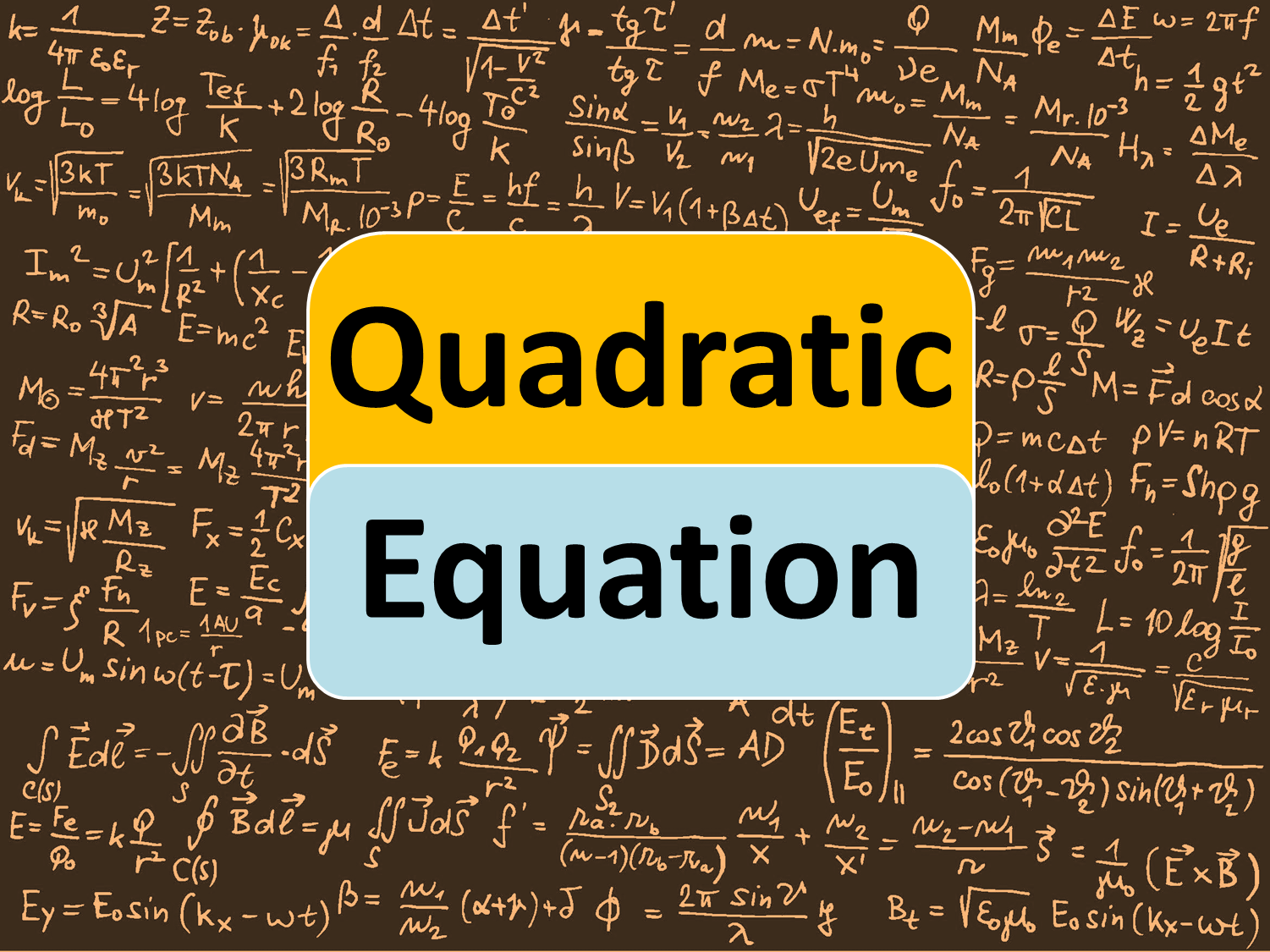Course Includes -

• Introduction Tutorial & 3 Methods of Quadratic Equations

• Special Questions Session + 100+ Previous Year Questions

• 100+ Practice Questions - Easy, Moderate and Hard Level Questions

• Time Saving Tricks and Tips, Preparation Stuff and Revision Class

Course Beneficial For - All Upcoming Competitive Examinations 2021

₹ 20.00
₹ 20.00
Last Update 03/16/2021 02:34 Hours 1
Key Features :
• Course Validity 1 Year
• Short tricks
• Strong -Weak Areas Analysis
• Bi-lingual
• Latest Syllabus 2022-23
• Speed, Accuracy Boosters
• Revision Class
• 100+ Practice Questions

• Introduction To Quadratic Equations - 0
• Free preview
• Factorization Sridharacharya and Square Methods - 0
• The 3 Methods - Factorization, Sridharacharya and Squaring.
• Practice Questions on Factorization
• Practice Questions on Shridharacharya Method
• Practice Questions - Completing the Square Method.
• Quadratic Equations - Special Questions Session | Must Practice - 0
• Quadratic Equation - Special Question 1
• Quadratic Equation - Special Question 2
• Quadratic Equation - Special Question 3
• Quadratic Equation - Special Question 4
• Quadratic Equation - Special Question 5
• Quadratic Equation - Special Question 6
• Quadratic Equation - Special Question 7
• Quadratic Equation - Special Question 8
• Quadratic Equation - Special Question 9
• Quadratic Equation - Special Question 10
• Quadratic Equation - Special Question 11
• Quadratic Equation Quiz - Easy to Hard Level - 0
• Quadratic Equation Quiz - Easy Level
• Quadratic Equation Quiz - Moderate Level
• Quadratic Equation Quiz - Hard Level
• Important Question Session - 0
• Important Question Session
• MCQs With Solution - PDF
• Quadratic Equations - Short Tricks - 0
• Let's Know Magical Short Tricks...!!!